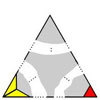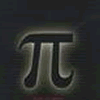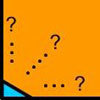Search by Topic

Resources tagged with Rational and irrational numbers similar to Eyes Down:

Filter by: Content type:
Age range:
Challenge level:

There are 20 results

Broad Topics > Numbers and the Number System > Rational and irrational numbersRationals Between...

Age 14 to 16 Challenge Level:

What fractions can you find between the square roots of 65 and 67?The Clue Is in the Question

Age 16 to 18 Challenge Level:

Starting with one of the mini-challenges, how many of the other mini-challenges will you invent for yourself?Impossible Triangles?

Age 16 to 18 Challenge Level:

Which of these triangular jigsaws are impossible to finish?Approximations, Euclid's Algorithm & Continued Fractions

Age 16 to 18

This article sets some puzzles and describes how Euclid's algorithm and continued fractions are related.The Root Cause

Age 16 to 18 Challenge Level:

Prove that if a is a natural number and the square root of a is rational, then it is a square number (an integer n^2 for some integer n.)Irrational Arithmagons

Age 16 to 18 Challenge Level:

Can you work out the irrational numbers that belong in the circles to make the multiplication arithmagon correct?Rational Roots

Age 16 to 18 Challenge Level:

Given that a, b and c are natural numbers show that if sqrt a+sqrt b is rational then it is a natural number. Extend this to 3 variables.Age 16 to 18 Short Challenge Level:

Can you work out where the blue-and-red brick roads end?Rational Round

Age 16 to 18 Challenge Level:

Show that there are infinitely many rational points on the unit circle and no rational points on the circle x^2+y^2=3.What Are Numbers?

Age 7 to 18

Ranging from kindergarten mathematics to the fringe of research this informal article paints the big picture of number in a non technical way suitable for primary teachers and older students.Continued Fractions II

Age 16 to 18

In this article we show that every whole number can be written as a continued fraction of the form k/(1+k/(1+k/...)).An Introduction to Proof by Contradiction

Age 14 to 18

An introduction to proof by contradiction, a powerful method of mathematical proof.The Square Hole

Age 14 to 16 Challenge Level:

If the yellow equilateral triangle is taken as the unit for area, what size is the hole ?An Introduction to Irrational Numbers

Age 14 to 18

Tim Rowland introduces irrational numbersProof Sorter - the Square Root of 2 Is Irrational

Age 16 to 18 Challenge Level:

Try this interactivity to familiarise yourself with the proof that the square root of 2 is irrational. Sort the steps of the proof into the correct order.Equal Equilateral Triangles

Age 14 to 16 Challenge Level:

Using the interactivity, can you make a regular hexagon from yellow triangles the same size as a regular hexagon made from green triangles ?Be Reasonable

Age 16 to 18 Challenge Level:

Prove that sqrt2, sqrt3 and sqrt5 cannot be terms of ANY arithmetic progression.Impossible Square?

Age 16 to 18 Challenge Level:

Can you make a square from these triangles?Good Approximations

Age 16 to 18 Challenge Level:

Solve quadratic equations and use continued fractions to find rational approximations to irrational numbers.Spirostars

Age 16 to 18 Challenge Level:

A spiropath is a sequence of connected line segments end to end taking different directions. The same spiropath is iterated. When does it cycle and when does it go on indefinitely?